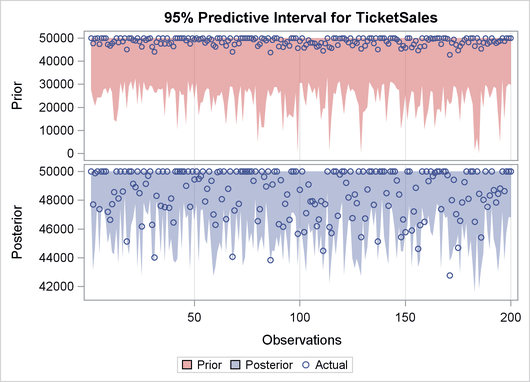The QLIM Procedure

Example 22.8 Bayesian Modeling

This example illustrates how to use the QLIM procedure to perform Bayesian analysis. The generated data mimic a hypothetical scenario in which you study the number of tickets sold for a sports event given the probability of the hosting team winning and the price of the tickets. The following statements create the dataset:

```
title1 'Bayesian Analysis';

ods graphics on;

data test;
do i=1 to 200;
e1 = rannor(8726)*2000;
WinChance = ranuni(8772);
Price = 10+ranexp(8773)*4;
y = 48000 + 5000*WinChance - 100 * price +  e1;
if y>50000 then TicketSales = 50000;
if y<=50000 then TicketSales = y;
output;
end;
keep WinChance price  y TicketSales;
run;

```

The following statements perform Bayesian analysis of a Tobit model:

```proc qlim data=test plots(prior)=all;
model TicketSales = WinChance price;
endogenous TicketSales ~ censored(lb=0 ub= 50000);
prior intercept~normal(mean=48000);
prior WinChance~normal(mean=5000);
prior Price~normal(mean=-100);
bayes NBI=10000 NMC=30000 THIN=1 ntrds=1 DIAG=ALL STATS=ALL seed=2;
run;
```

Output 22.8.1 shows the results from the maximum likelihood estimation and the Bayesian analysis with diffuse prior of this Tobit model.

Output 22.8.1: Bayesian Tobit Model

 Bayesian Analysis

The QLIM Procedure

Parameter Estimates
Parameter DF Estimate Standard
Error
t Value Approx
Pr > |t|
Intercept 1 48119 623.565045 77.17 <.0001
WinChance 1 5242.083501 559.151222 9.38 <.0001
Price 1 -106.731665 40.660795 -2.62 0.0087
_Sigma 1 1939.607206 134.348772 14.44 <.0001

Posterior Summaries
Parameter N Mean Standard
Deviation
Percentiles
25% 50% 75%
Intercept 30000 48109.4 535.0 47750.5 48102.6 48460.1
WinChance 30000 5212.9 483.4 4878.8 5205.2 5533.0
Price 30000 -104.7 36.5224 -128.6 -104.2 -79.4191
_Sigma 30000 1950.9 132.9 1858.4 1945.0 2034.0

Output 22.8.2 depicts a graphical representation of MLE, prior, and posterior distributions.

Output 22.8.2: Predictive Analysis by Observation Number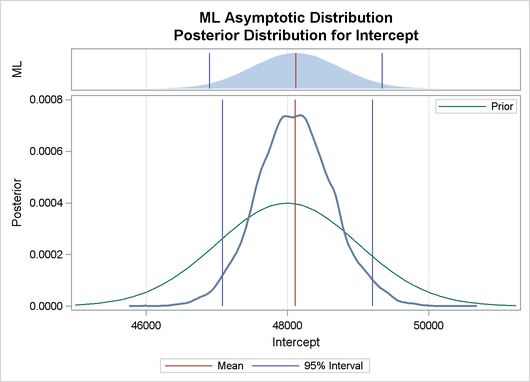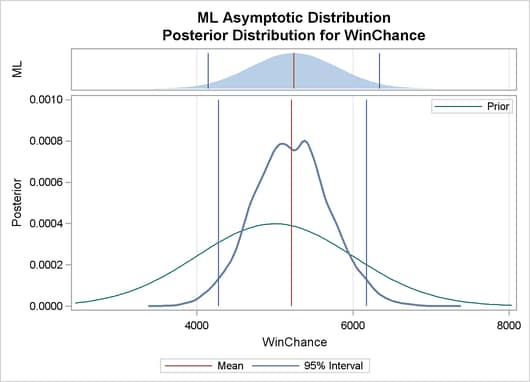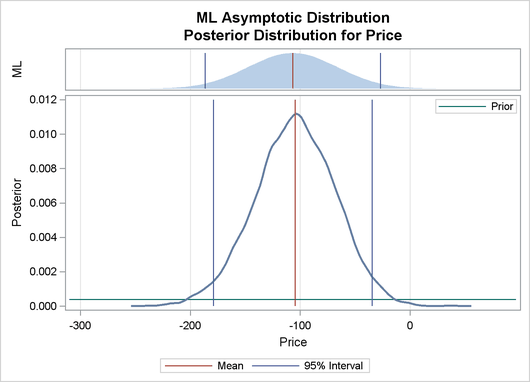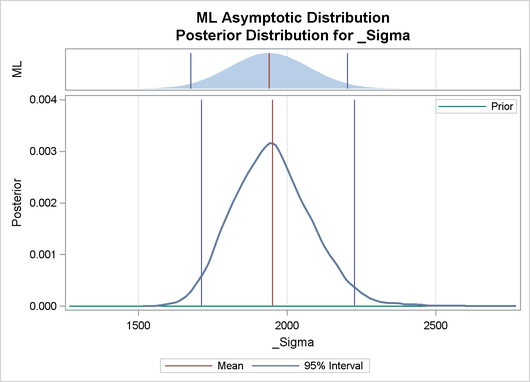The validity of the MCMC sampling phase can be monitored with Output 22.8.3.

Output 22.8.3: Predictive Analysis by Observation Number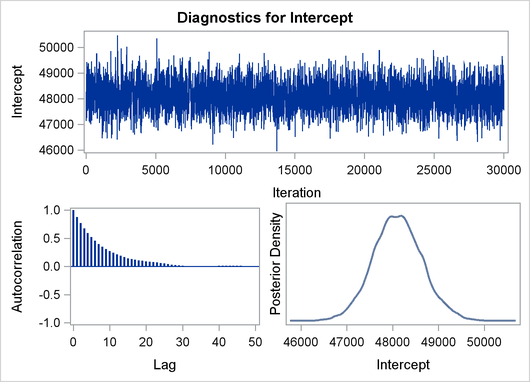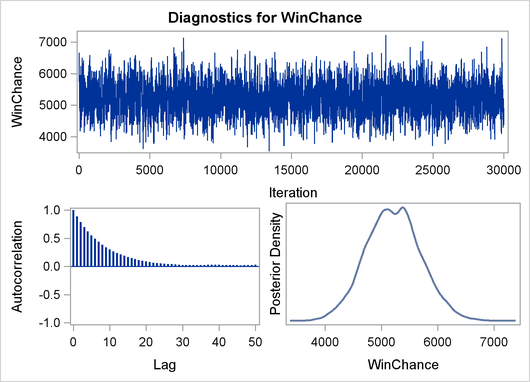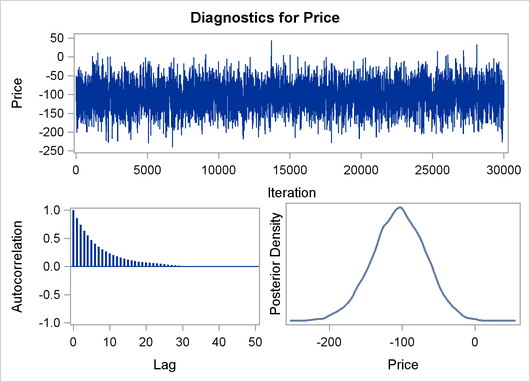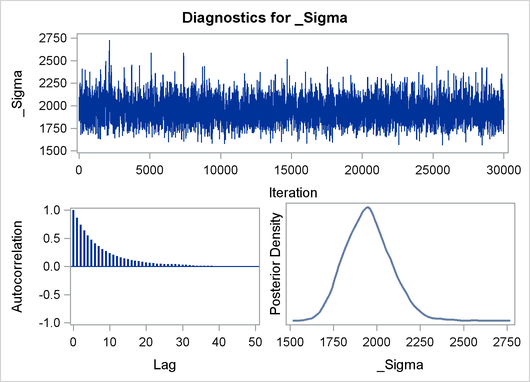Finally the prior and the posterior predictive analyses are represented in Output 22.8.4

Output 22.8.4: Predictive Analysis by Observation Number